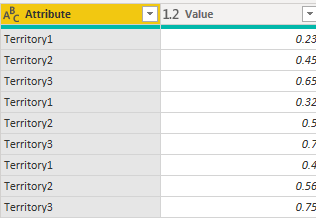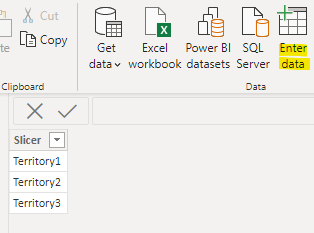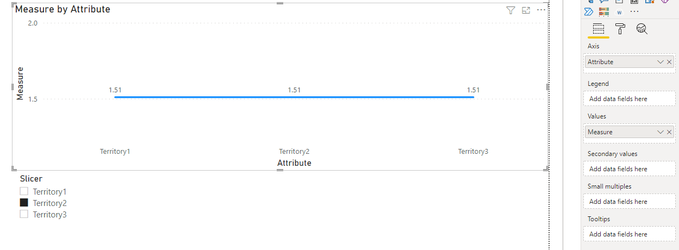cancel
Showing results for
Did you mean:Helper V

## Powerbi slicer

•@abgnfirdaus  did you get answer for this? Even I'm trying I couldn't.

1 ACCEPTED SOLUTIONCommunity Support

Hi  @AtchayaP ,

Here are the steps you can follow：

1. Select three columns in Power Query – Unpivot Columns.Result：2. Use Enter data to create data.3. Create measure.

``````Measure =
var _select=SELECTEDVALUE('Slicer_table'[Slicer])
return
IF(
_select ="Territory2",
SUMX(FILTER(ALL('Table'),'Table'[Attribute]="Territory2"),[Value]),SUMX(FILTER(ALL('Table'),'Table'[Attribute]=MAX('Table'[Attribute])),[Value]))``````

4. Result:Best Regards,

Liu Yang

If this post helps, then please consider Accept it as the solution to help the other members find it more quicklyCommunity Support

Hi  @AtchayaP ,

Here are the steps you can follow：

1. Select three columns in Power Query – Unpivot Columns.Result：2. Use Enter data to create data.3. Create measure.

``````Measure =
var _select=SELECTEDVALUE('Slicer_table'[Slicer])
return
IF(
_select ="Territory2",
SUMX(FILTER(ALL('Table'),'Table'[Attribute]="Territory2"),[Value]),SUMX(FILTER(ALL('Table'),'Table'[Attribute]=MAX('Table'[Attribute])),[Value]))``````

4. Result:Best Regards,

Liu Yang

If this post helps, then please consider Accept it as the solution to help the other members find it more quickly